# Overview

This is the fourth blog post of my Finaical Analysis in Python series.

This blog series covers topics like the following:

• how to visualize the long-term investment plan
• backtest dollar-cost averaging strategy for long-term investment
• backtest value averaging strategy for long-term investment
• compare different investment strategies for short-term investment

# Disclaimer

Investing money into anything is often involved with risk. Please do your own research before investing and be responsible for your own investment decisions.

I am just learning investment on my own and want to share some codes that I have written that might be useful for others.

The content here is only for informational purpose (instead of taking them as professional investment advice).

# Introduction

Imagine that we have a fixed amount of initial investment and we want to set up a short-term investment plan.

We can either decide to invest all at once into the stock market at the beginning of each year, or apply the dollar-cost averaging or value averaging on a monthly or quarterly basis.

Which method could maximize our investment return?

Let’s do some backtests using the SP500 index performance data.

# Import libraries
import datetime

from matplotlib import pyplot as plt
import pandas as pd
from pandas_datareader import data as pdr

plt.style.use('bmh')

# Get historical data
startdate = datetime.datetime(1988, 1, 1)
enddate = datetime.datetime(2021, 1, 1)

# SP 500 total return index
SP = pdr.get_data_yahoo('^SP500TR', start=startdate, end=enddate)
SP['date'] = SP.index


# Compare all-in with dollar cost averaging

Let’s first assume that the timeframe for the short-term investment plan is one year and the investment should start at the beginning of each year. Hence. each year between 1980 and 2020 is an individual sample.

In this section, I will compare the annual return rates for these investment plans:

• Plan 1: All-in approach at the beginning of a year
• Plan 2: Divide the initial investment into 12 folds and invest at the beginning of each month
• Plan 3: Divide the initial investment into 4 folds and invest at the beginning of each quarter
# Plan 1: All-in
# Group by year
SP['date'] = SP.index
SP['year'] = SP['date'].apply(lambda d: f'{d.year}')
df['return_next_y'] = df['return'].shift(-1)

# Get a feeling of the return rate per year
df['return_next_y'].hist(bins=20);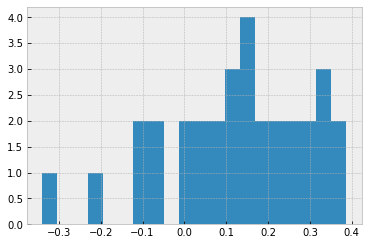# Plan 2: Monthly dollar-cost averaging
# Group by month
SP['month'] = SP['date'].apply(lambda d: f'{d.month:02d}')
df_m = SP[['year', 'month', 'Adj Close']].groupby(['year','month']).first()
df_m['return_next'] = df_m['return'].shift(-1)

# Plan 3: Quarterly dollar-cost averaging
# Group by quarter
SP['quarter'] = SP['month'].apply(lambda m: int(m)//4 + 1)
df_q = SP[['year', 'quarter', 'Adj Close']].groupby(['year','quarter']).first()
df_q['return_next'] = df_q['return'].shift(-1)


Here is how the dataframe for the quarterly dollar-cost averaging looks like:

df_q.head()

year quarter Adj Close return return_next
1988 1 256.019989 NaN 0.009335
2 258.410004 0.009335 0.074881
3 277.760010 0.074881 0.015085
4 281.950012 0.015085 0.013123
1989 1 285.649994 0.013123 0.085979
# Function for calcuting dollar-cost averaging returns
def calc_return_over_periods_list(
investment_per_period_list: list,
return_per_period_list: list,
n_period: int,
print_values: bool = False) -> float:

"""
This function calculates the overall rate of return of the dollar-cost averaging strategy.
:param investment_per_period_list: Investment value at the beginning of each period.
:param return_per_period_list: Historical return rate per period.
:param n_period: number of periods for calculating the overall return rate.
:param print_values: Whether to display the value each period and overall return rate or not
:return: A dataframe that contains all the relevant info
"""

value = 0

for i in range(n_period):
value += investment_per_period_list[i]
value = value * (1 + return_per_period_list[i])
if print_values:
print(f'Peorid {i}: value = {round(value, 2)}')

return_rate = value/sum(investment_per_period_list) - 1

if print_values:
print(f'The overall return rate is {round(return_rate*100,2)}%')

return return_rate


Here is how the function works for calculating the overall return for a dollar-cost averaging strategy:

# Assuming investing 300 dollars at the beginning of each quarter
calc_return_over_periods_list(
investment_per_period_list = *4,
return_per_period_list = df_q.loc['2019','return_next'].tolist(),
n_period=4,
print_values=True)


Output:

Peorid 0: value = 344.45
Peorid 1: value = 668.05
Peorid 2: value = 1027.87
Peorid 3: value = 1391.54

The overall return rate is 15.96%.


# Join dollar-cost averaging results together into one dataframe
df = df.drop(columns='return')

for year in SP.year.unique()[:-1]:
# for monthly dollar-cost averaging annual return
df.loc[f'{int(year)}', 'return_next_m'] = (
calc_return_over_periods_list(
*12,
df_m.loc[(f'{year}'),'return_next'].tolist(),
12)
)

# for quarterly dollar-cost averaging annual return
df.loc[f'{int(year)}', 'return_next_q'] = (
calc_return_over_periods_list(
*4,
df_q.loc[(f'{year}'),'return_next'].tolist(),
4)
)

df.round(3)

year Adj Close return_next_y return_next_m return_next_q
1988 256.02 0.116 0.059 0.066
1989 285.65 0.352 0.152 0.172
1990 386.16 -0.059 -0.008 -0.031
1991 363.44 0.320 0.137 0.165
1992 479.84 0.075 0.064 0.057
1993 516.00 0.099 0.047 0.053
1994 567.10 0.015 0.015 0.029
1995 575.52 0.387 0.188 0.198
1996 798.22 0.214 0.121 0.120
1997 969.05 0.347 0.151 0.172
1998 1305.04 0.279 0.159 0.139
1999 1668.52 0.200 0.118 0.120
2000 2002.11 -0.108 -0.101 -0.094
2001 1785.86 -0.088 -0.026 -0.023
2002 1628.51 -0.200 -0.078 -0.097
2003 1303.17 0.242 0.179 0.182
2004 1618.05 0.103 0.074 0.071
2005 1784.96 0.075 0.062 0.053
2006 1918.96 0.138 0.096 0.096
2007 2183.92 0.041 -0.008 0.013
2008 2273.41 -0.341 -0.229 -0.189
2009 1499.17 0.245 0.249 0.207
2010 1867.06 0.145 0.141 0.109
2011 2138.30 0.025 0.018 0.007
2012 2192.40 0.172 0.079 0.084
2013 2568.55 0.280 0.145 0.143
2014 3286.69 0.147 0.087 0.085
2015 3768.68 -0.001 -0.009 -0.020
2016 3763.99 0.146 0.094 0.084
2017 4315.08 0.218 0.121 0.124
2018 5256.28 -0.051 -0.078 -0.066
2019 4990.56 0.324 0.150 0.160
2020 6609.29 NaN NaN NaN

With the dataframe above, we can easily compare the performance of these three short-term investment plans:

# Return_next_y is the annual return for all-in method
# Return_next_m is the annual return of the monthly dollar cost averaging method
# Return_next_q is the annual return of the quarterly dollar cost averaging method
df[['return_next_y','return_next_m','return_next_q']].describe().round(3)

return_next_y return_next_m return_next_q
count 32.000 32.000 32.000
mean 0.121 0.068 0.068
std 0.169 0.099 0.096
min -0.341 -0.229 -0.189
25% 0.023 0.009 0.012
50% 0.142 0.083 0.084
75% 0.243 0.142 0.140
max 0.387 0.249 0.207
plt.figure(figsize=(15,5))
plt.plot(df['return_next_y'], label='all-in')
plt.plot(df['return_next_m'], label='dollar-cost (monthly)')
plt.plot(df['return_next_q'], label='dollar-cost (quarterly)')
plt.axhline(y=0, color='r', linestyle='-')
plt.xlabel('year')
plt.ylabel('return rate')
plt.xticks(rotation=90)
plt.title('Annual return rate of the all-in vs. dollar-cost average methods')
plt.legend()
plt.show()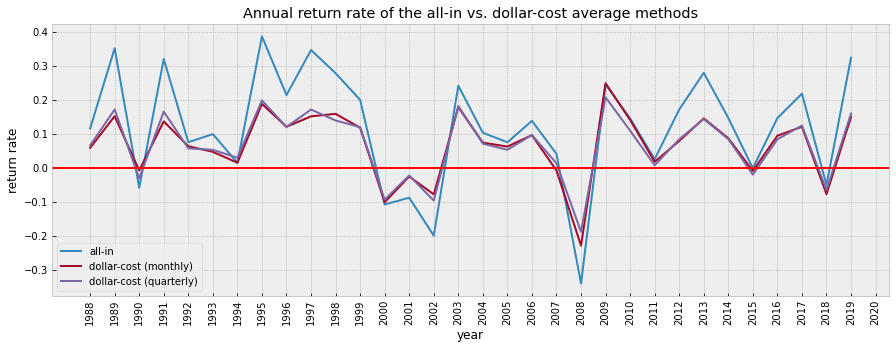## Observation

Given that you already have a fixed amount of initial investment and you want to invest it into an ETF based on SP500, all-in method performed better than both monthly and quarterly dollar-cost averaging methods in most of the past years.

This is because SP500 has a tendency of increasing in most of the past years; and the all-in method maximized the gain.

However, dollar-cost averaging method helps to reduce loss in a bear market.

It seems that only in the following years SP500 has a negative year-over-year return.

list(df.loc[df['return_next_y']<=0,:].index)

['1990', '2000', '2001', '2002', '2008', '2015', '2018']


The bear markets does not seem to last long using the SP 500 index (usually less than 2 years).

So, it might be a good time to start investing if the last year was a bear market.

If it is already a bull market, you can decide whether you still want to risk it with the all-in method.

If it is already a bear market, it might be a good time to start investing.

# Compare with value averaging

In the last notebook, we already discussed the method of value averaging for long term investment, and we assumed that it might work for short term investment.

Let’s check the annual return of the value averaging method here:

# This is an adjusted version of the function that we have introduced in the last notebook for value averaging
def calc_value_averaging_return(df: pd.DataFrame, value_per_period: float = 1.0,
increase_investment_per_period: float = 0.0) -> float:
"""
This function calculates the overall return rate of the value averaging strategy.
:param df: Original dataframe that contains the price at the beginning of each period
:param value_per_period: Investment value per period
:param increase_investment_per_period: Increase the investment by x each period
:return: overall return rate (might be negative if the total withdraw amount is too much)
"""
df_va = pd.DataFrame.copy(df)
value = value_per_period
df_va.loc[0,'target_value'] = value
df_va.loc[0,'should_invest_or_withdraw'] = value
df_va.loc[0,'total_invest_or_withdraw'] = value

for i in range(1,len(df_va.index)):
value += value_per_period*((1 + increase_investment_per_period)**i)
df_va.loc[i,'target_value'] = value
df_va.loc[i,'total_invest_or_withdraw'] = df_va.loc[i-1,'total_invest_or_withdraw'] + df_va.loc[i,'should_invest_or_withdraw']

return df_va.loc[len(df_va.index)-1,'target_value']/df_va.loc[len(df_va.index)-1,'total_invest_or_withdraw']-1

# Assuming fixed investment value each year
value_per_year = 1200
value_per_quarter = value_per_year/4
value_per_month = value_per_year/12

### Join results together into one dataframe
for year in SP.year.unique()[:-1]:
df_va_m_sample = df_va_m.loc[df_va_m['year']==year,:].reset_index()
df_va_q_sample = df_va_q.loc[df_va_q['year']==year,:].reset_index()
annual_return_va_m = calc_value_averaging_return(df_va_m_sample, value_per_month)
annual_return_va_q = calc_value_averaging_return(df_va_q_sample, value_per_quarter)
df.loc[f'{int(year)}', 'return_va_m'] = annual_return_va_m
df.loc[f'{int(year)}', 'return_va_q'] = annual_return_va_q

# Join value averaging with dollar cost averaging results
df.round(3)

year Adj Close return_next_y return_next_m return_next_q return_va_m return_va_q
1988 256.02 0.116 0.059 0.066 0.049 0.054
1989 285.65 0.352 0.152 0.172 0.128 0.152
1990 386.16 -0.059 -0.008 -0.031 -0.011 -0.037
1991 363.44 0.320 0.137 0.165 0.037 0.065
1992 479.84 0.075 0.064 0.057 0.052 0.045
1993 516.00 0.099 0.047 0.053 0.038 0.044
1994 567.10 0.015 0.015 0.029 -0.008 0.005
1995 575.52 0.387 0.188 0.198 0.171 0.188
1996 798.22 0.214 0.121 0.120 0.165 0.170
1997 969.05 0.347 0.151 0.172 0.161 0.194
1998 1305.04 0.279 0.159 0.139 0.128 0.094
1999 1668.52 0.200 0.118 0.120 0.080 0.078
2000 2002.11 -0.108 -0.101 -0.094 -0.072 -0.068
2001 1785.86 -0.088 -0.026 -0.023 -0.040 -0.041
2002 1628.51 -0.200 -0.078 -0.097 -0.052 -0.062
2003 1303.17 0.242 0.179 0.182 0.145 0.156
2004 1618.05 0.103 0.074 0.071 0.067 0.064
2005 1784.96 0.075 0.062 0.053 0.062 0.050
2006 1918.96 0.138 0.096 0.096 0.083 0.086
2007 2183.92 0.041 -0.008 0.013 0.009 0.029
2008 2273.41 -0.341 -0.229 -0.189 -0.275 -0.236
2009 1499.17 0.245 0.249 0.207 0.255 0.216
2010 1867.06 0.145 0.141 0.109 0.093 0.054
2011 2138.30 0.025 0.018 0.007 -0.001 -0.019
2012 2192.40 0.172 0.079 0.084 0.041 0.044
2013 2568.55 0.280 0.145 0.143 0.134 0.131
2014 3286.69 0.147 0.087 0.085 0.088 0.085
2015 3768.68 -0.001 -0.009 -0.020 0.040 0.022
2016 3763.99 0.146 0.094 0.084 0.063 0.052
2017 4315.08 0.218 0.121 0.124 0.102 0.107
2018 5256.28 -0.051 -0.078 -0.066 0.027 0.039
2019 4990.56 0.324 0.150 0.160 0.104 0.113
2020 6609.29 NaN NaN NaN NaN NaN
plt.figure(figsize=(15,5))
plt.plot(df['return_next_y'], label='all-in')
plt.plot(df['return_va_m'], label='value-averaging (monthly)')
# plt.plot(df['return_va_q'], label='value-averaging (quarterly)')
plt.plot(df['return_next_m'], label='dollar-cost (monthly)')
# plt.plot(df['return_next_q'], label='dollar-cost (quarterly)')
plt.axhline(y=0, color='r', linestyle='-')
plt.xlabel('year')
plt.ylabel('return rate')
plt.xticks(rotation=90)
plt.title('Annual return rate of the all-in, monthly dollar-cost averaging, and monthly value averaging methods')
plt.legend()
plt.show()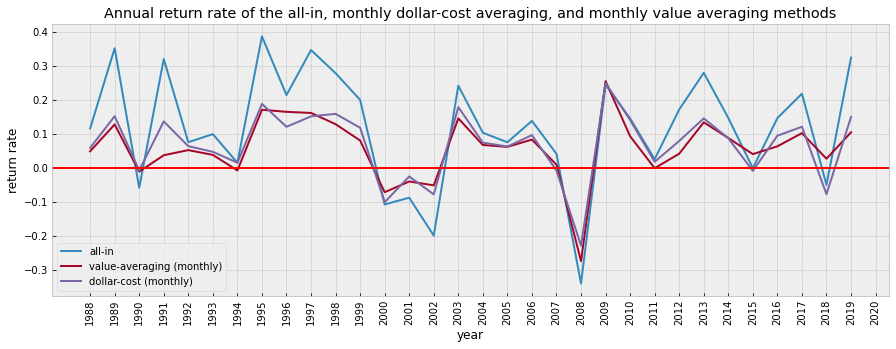def compare_strategy(df, str1, str2, str1_name, str2_name):
print(f'number of times {str1_name} is better than {str2_name}: {df.loc[df[str1] > df[str2],:].shape}')
print(f'number of times {str1_name} is equal to {str2_name}: {df.loc[df[str1] == df[str2],:].shape}')
print(f'number of times {str1_name} is worse than {str2_name}: {df.loc[df[str1] < df[str2],:].shape}')


compare_strategy(df, 'return_va_m', 'return_next_m', 'monthly value-averaging', 'monthly dollar-cost-averaging')


Output:

number of times monthly value-averaging is better than monthly dollar-cost-averaging: 9
number of times monthly value-averaging is equal to monthly dollar-cost-averaging: 0
number of times monthly value-averaging is worse than monthly dollar-cost-averaging: 23


compare_strategy(df, 'return_next_y', 'return_next_m', 'all-in', 'monthly dollar-cost-averaging')


Output:

number of times all-in is better than monthly dollar-cost-averaging: 26
number of times all-in is equal to monthly dollar-cost-averaging: 0
number of times all-in is worse than monthly dollar-cost-averaging: 6


## Observation

It seems that the annual return of the dollar-cost averaging and value averaging did not differ much, even though the monthly value averaging method was worse than the monthly dollar-cost-averaging in most years.

Again, all-in method brings the most gain but also involves the most risk.

# What if we use a multi-year timeframe?

The test below involves a multiple year short-term investment plan. The rate of return below indicates the overall rate of return of investing for x years starting from a certain year.

Let’s see if using dollar cost averaging or value averaging helps to improve the peformance.

SP

Date High Low Open Close Volume Adj Close date year month quarter
1988-01-04 256.019989 256.019989 256.019989 256.019989 0 256.019989 1988-01-04 1988 01 1
1988-01-05 258.769989 258.769989 258.769989 258.769989 0 258.769989 1988-01-05 1988 01 1
1988-01-06 259.029999 259.029999 259.029999 259.029999 0 259.029999 1988-01-06 1988 01 1
1988-01-07 261.209991 261.209991 261.209991 261.209991 0 261.209991 1988-01-07 1988 01 1
1988-01-08 243.550003 243.550003 243.550003 243.550003 0 243.550003 1988-01-08 1988 01 1
... ... ... ... ... ... ... ... ... ... ...
2020-09-21 6757.350098 6641.330078 6757.350098 6748.080078 0 6748.080078 2020-09-21 2020 09 3
2020-09-22 6828.669922 6727.319824 6778.330078 6819.080078 0 6819.080078 2020-09-22 2020 09 3
2020-09-23 6835.209961 6649.240234 6828.919922 6657.819824 0 6657.819824 2020-09-23 2020 09 3
2020-09-24 6744.000000 6602.359863 6635.979980 6678.040039 0 6678.040039 2020-09-24 2020 09 3
2020-09-25 6802.140137 6642.040039 6657.830078 6784.950195 0 6784.950195 2020-09-25 2020 09 3

8250 rows 10 columns

def compare_returns(SP, n_years):
"""
This function aims to compare 5 n-year short-term investment plans based on a certain ETF/stock's performance data.
:param SP: Original dataframe that contains a certain ETF/stock's performance data
:param n_years: Number of years set for the short-term investment plan
:return: A dataframe that contains the overall return for the 5 plans
"""
test_months = 12*n_years
test_quarters = 4*n_years

# Compute all in return
df['return_next'] = df['return'].shift(-n_years)
df = df.drop(columns='return')

for year in SP.year.unique()[:-n_years]:
# Compute monthly dollar cost averaging return
df.loc[f'{int(year)}', 'return_next_m'] = (
_calc_return_over_periods_list_simplified(
*test_months,
df_m.loc[(f'{year}'):(f'{int(year) + n_years - 1}'),'return_next'].tolist(),
test_months)
)

# Compute quarterly dollar cost averaging return
df.loc[f'{int(year)}', 'return_next_q'] = (
_calc_return_over_periods_list_simplified(
*test_quarters,
df_q.loc[(f'{year}'):(f'{int(year) + n_years - 1}'),'return_next'].tolist(),
test_quarters)
)

# Compute value averaging returns

### Join results together into one dataframe
for year in SP.year.unique()[:-n_years]:
df_va_m_sample = df_va_m.loc[(df_va_m['year']>=year) &
(df_va_m['year']<=str(int(year) + n_years - 1)),:].reset_index()
df_va_q_sample = df_va_q.loc[(df_va_q['year']>=year) &
(df_va_q['year']<=str(int(year) + n_years - 1)),:].reset_index()
annual_return_va_m = _calc_value_averaging_return_simplified(df_va_m_sample)
annual_return_va_q = _calc_value_averaging_return_simplified(df_va_q_sample)
df.loc[f'{int(year)}', 'return_va_m'] = annual_return_va_m
df.loc[f'{int(year)}', 'return_va_q'] = annual_return_va_q

return df

def _calc_return_over_periods_list_simplified(
investment_per_period_list: list,
return_per_period_list: list,
n_period: int,
print_values=False) -> float:

value = 0
for i in range(n_period):
value += investment_per_period_list[i]
value = value * (1 + return_per_period_list[i])
return_rate = value/sum(investment_per_period_list) - 1

return return_rate

def _calc_value_averaging_return_simplified(df_va, value_per_period=1):

df_va.loc[0,'total_value'] = value_per_period
df_va.loc[0,'should_invest'] = value_per_period
df_va.loc[0,'total_invest'] = value_per_period

for i in range(1,len(df_va.index)):
df_va.loc[i,'total_value'] = value_per_period*(i+1)
df_va.loc[i,'total_invest'] = df_va.loc[i-1,'total_invest'] + df_va.loc[i,'should_invest']

return df_va.loc[len(df_va.index)-1,'total_value']/df_va.loc[len(df_va.index)-1,'total_invest']-1

def plot_changes(df):
plt.figure(figsize=(15,5))
plt.plot(df['return_next'], label='all-in')
plt.plot(df['return_va_m'], label='value-averaging (monthly)')
# plt.plot(df['return_va_q'], label='value-averaging (quarterly)')
plt.plot(df['return_next_m'], label='dollar-cost (monthly)')
# plt.plot(df['return_next_q'], label='dollar-cost (quarterly)')
plt.axhline(y=0, color='r', linestyle='-')
plt.xlabel('year')
plt.ylabel('return rate')
plt.xticks(rotation=90)
plt.title('Annual return rate of the all-in, monthly dollar-cost averaging, and monthly value averaging methods')
plt.legend()
plt.show()


# Assuming we are setting up a 2 year plan and then compare the return
test_years = 2
df_2 = compare_returns(SP, test_years)

df_2.round(3)

year Adj Close return_next return_next_m return_next_q return_va_m return_va_q
1988 256.02 0.508 0.291 0.306 0.296 0.322
1989 285.65 0.272 0.038 0.036 0.039 0.030
1990 386.16 0.243 0.223 0.222 0.127 0.130
1991 363.44 0.420 0.143 0.155 0.138 0.152
1992 479.84 0.182 0.108 0.107 0.102 0.102
1993 516.00 0.115 0.039 0.049 0.017 0.026
1994 567.10 0.408 0.298 0.313 0.308 0.340
1995 575.52 0.684 0.281 0.287 0.367 0.390
1996 798.22 0.635 0.330 0.340 0.393 0.437
1997 969.05 0.722 0.315 0.318 0.312 0.300
1998 1305.04 0.534 0.254 0.243 0.239 0.212
1999 1668.52 0.070 -0.052 -0.048 -0.020 -0.021
2000 2002.11 -0.187 -0.103 -0.098 -0.105 -0.109
2001 1785.86 -0.270 -0.149 -0.157 -0.114 -0.112
2002 1628.51 -0.006 0.161 0.152 0.133 0.136
2003 1303.17 0.370 0.187 0.187 0.189 0.194
2004 1618.05 0.186 0.109 0.102 0.113 0.104
2005 1784.96 0.224 0.153 0.147 0.146 0.144
2006 1918.96 0.185 0.066 0.077 0.088 0.099
2007 2183.92 -0.314 -0.288 -0.260 -0.310 -0.277
2008 2273.41 -0.179 0.104 0.109 0.116 0.143
2009 1499.17 0.426 0.286 0.246 0.258 0.204
2010 1867.06 0.174 0.094 0.072 0.082 0.046
2011 2138.30 0.201 0.136 0.132 0.108 0.097
2012 2192.40 0.499 0.263 0.265 0.275 0.278
2013 2568.55 0.467 0.200 0.198 0.217 0.212
2014 3286.69 0.145 0.038 0.032 0.095 0.078
2015 3768.68 0.145 0.115 0.104 0.091 0.074
2016 3763.99 0.396 0.227 0.222 0.222 0.219
2017 4315.08 0.157 -0.007 0.001 0.111 0.120
2018 5256.28 0.257 0.185 0.199 0.154 0.168
2019 4990.56 NaN NaN NaN NaN NaN
2020 6609.29 NaN NaN NaN NaN NaN
plot_changes(df_2)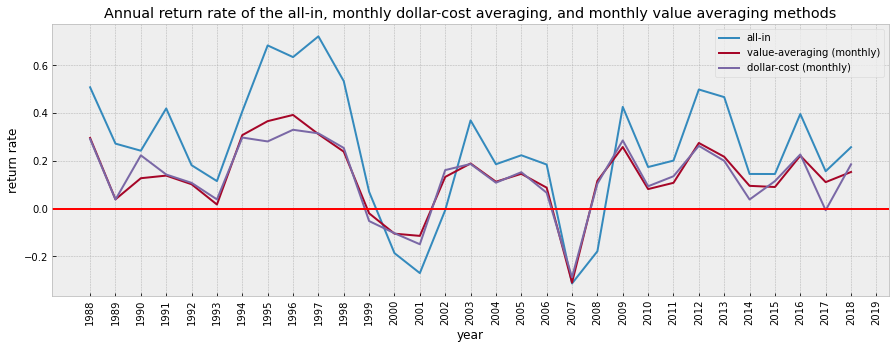compare_strategy(df_2, 'return_va_m', 'return_next_m', 'monthly value-averaging', 'monthly dollar-cost-averaging')


Output:

number of times monthly value-averaging is better than monthly dollar-cost-averaging: 15
number of times monthly value-averaging is equal to monthly dollar-cost-averaging: 0
number of times monthly value-averaging is worse than monthly dollar-cost-averaging: 16


compare_strategy(df_2, 'return_next', 'return_next_m', 'all-in', 'monthly dollar-cost-averaging')


Output:

number of times all-in is better than monthly dollar-cost-averaging: 26
number of times all-in is equal to monthly dollar-cost-averaging: 0
number of times all-in is worse than monthly dollar-cost-averaging: 5


# Assuming we are setting up a 3 year plan and then compare the return
test_years = 3
df_3 = compare_returns(SP, test_years)

plot_changes(df_3)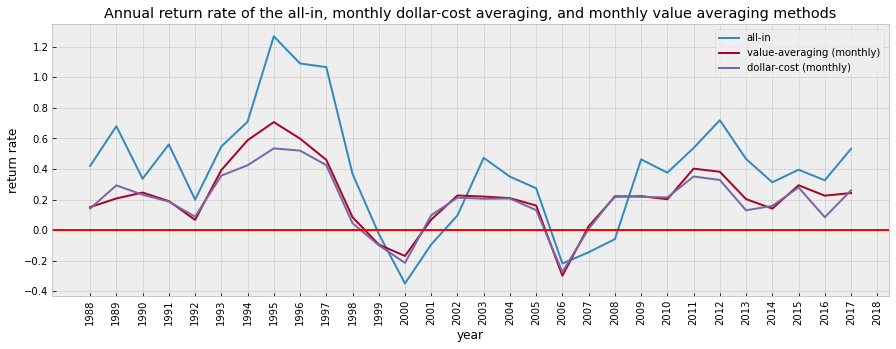compare_strategy(df_3, 'return_va_m', 'return_next_m', 'monthly value-averaging', 'monthly dollar-cost-averaging')


Output:

number of times monthly value-averaging is better than monthly dollar-cost-averaging: 22
number of times monthly value-averaging is equal to monthly dollar-cost-averaging: 0
number of times monthly value-averaging is worse than monthly dollar-cost-averaging: 8


compare_strategy(df_3, 'return_next', 'return_next_m', 'all-in', 'monthly dollar-cost-averaging')


Output:

number of times all-in is better than monthly dollar-cost-averaging: 25
number of times all-in is equal to monthly dollar-cost-averaging: 0
number of times all-in is worse than monthly dollar-cost-averaging: 5


# Assuming we are setting up a 4 year plan and then compare the return
test_years = 4
df_4 = compare_returns(SP, test_years)

plot_changes(df_4)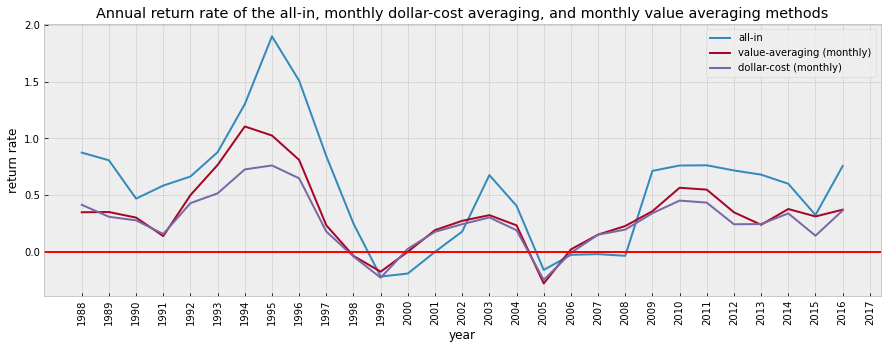compare_strategy(df_4, 'return_va_m', 'return_next_m', 'monthly value-averaging', 'monthly dollar-cost-averaging')


Output:

number of times monthly value-averaging is better than monthly dollar-cost-averaging: 24
number of times monthly value-averaging is equal to monthly dollar-cost-averaging: 0
number of times monthly value-averaging is worse than monthly dollar-cost-averaging: 5


compare_strategy(df_4, 'return_next', 'return_next_m', 'all-in', 'monthly dollar-cost-averaging')


Output:

number of times all-in is better than monthly dollar-cost-averaging: 23
number of times all-in is equal to monthly dollar-cost-averaging: 0
number of times all-in is worse than monthly dollar-cost-averaging: 6


# Conclusion

When we have a fixed amount of money, in most cases, using the all-in method performs the best, but it also involves higher risk.

The value averaging strategy did not differ much from the dollar cost averaging. When it is a one or two year short investment plan, the dollar cost averaging is more likely to perform better; if it is a three or four year investment plan, the value averaging strategy is more likely to perform better.

Given that the value averaging strategy is a bit tedious to implement, I think it is better to use either all-in or dollar-cost averaging for short term investment.

Please note that, in this notebook, I did not use the adjusted version of the value averaging method with the minimum and maximum thresholds of investment per period (as introduced in BP3 Plan 3). It might bring you some extra return if it is a longer term investment plan.